## Overview

• Loss functions (or cost/error functions) compute the distance between the current output of the algorithm and the expected output. It’s a method to evaluate how your algorithm models the data. Put informally, they are a window to your model’s heart.
• We will first look into typical modeling tasks and then go over common loss functions and their respective use cases. For ease of understanding, the loss functions have been segregated into classification and regression.

• Let’s consider a neural network setup with a CNN model, activation function (softmax or sigmoid), and a cross-entropy loss: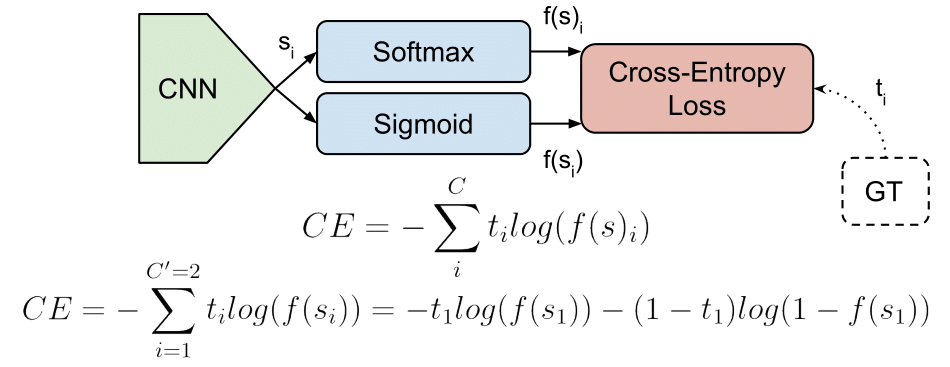### Binary classification

• In machine learning, binary classification is a supervised learning algorithm that categorizes new observations into one of two classes. The model has a single output (which is fed as input to the sigmoid function) in the range [0,1]. If the output > 0.5, then class 1 (positive class), else 0 (negative class).
• Typical binary classification problems include:
• Medical testing to determine if a patient has certain disease or not;
• Quality control in industry, deciding whether a specification has been met;
• In information retrieval, deciding whether a page should be in the result set of a search or not.

### Multi-Class Classification

• One-of-many classification. Each sample can belong to only one of $$C$$ classes. The model has $$C$$ output neurons that can be gathered in a scores vector $$s$$ (which is fed as input to the softmax function) . The target (ground truth) vector $$t$$ will be a one-hot vector with a positive (1) class and $$C-1$$ negative (0) classes.
• This task is treated as a single classification problem of samples in one of $$C$$ classes.

### Multi-Label Classification

• Each sample can belong to more than one class. The model has $$C$$ output neurons (similar to multi-class classification). The target vector $$t$$ can have more than a positive class, so it will be a multi-hot vector of 0s and 1s with $$C$$ dimensionality (as opposed to a one-hot vector in case of multi-class classification). This task is treated as $$C$$ independent binary $$\left(C^{\prime}=2, t^{\prime}=0\right. or \left.t^{\prime}=1\right)$$ classification problems, where each output neuron decides if a sample belongs to a class or not.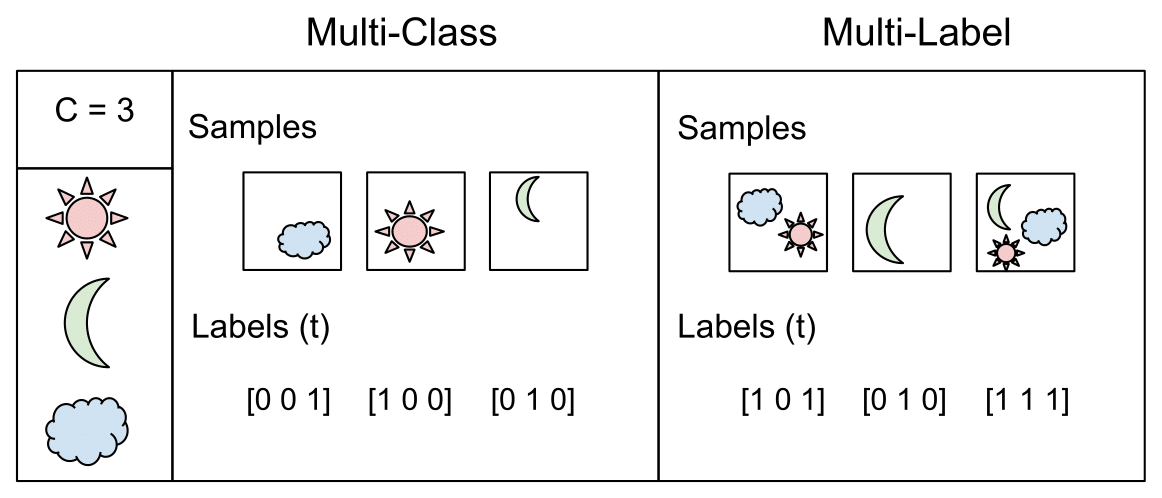## Output Activation Functions

• These functions are transformations we apply to vectors coming out from the model before the loss computation.

### Sigmoid

• The Sigmoid function is used for binary classification. It squashes a vector in the range (0, 1). It is applied independently to each element of $$s$$. It is also called the logistic function (since it is used in logistic regression for binary classification).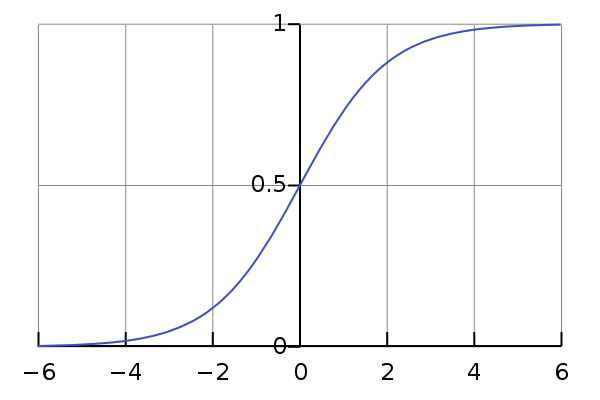$f(s_{i}) = \frac{1}{1 + e^{-s_{i}}}$

### Softmax

• The Softmax function is a generalization of the sigmoid function for multi-class classification. In other words, use sigmoid for binary classification and softmax for multiclass classification. Softmax is a function, not a loss. It squashes a vector in the range (0, 1) and all the resulting elements sum up to 1. It is applied to the output scores $$s$$. As elements represent a class, they can be interpreted as class probabilities.
• The Softmax function cannot be applied independently to each $$s_i$$, since it depends on all elements of $$\boldsymbol{s}$$. For a given class $$s_i$$, the Softmax function can be computed as:

$f(s)_i=\frac{e^{s_i}}{\sum_j^C e^{s_j}}$
• where $$s_j$$ are the scores inferred by the net for each class in $$C$$. Note that the Softmax activation for a class $$s_i$$ depends on all the scores in $$s$$.
• Activation functions are used to transform vectors before computing the loss in the training phase. In testing, when the loss is no longer applied, activation functions are also used to get the CNN outputs.

## Classification Loss Functions

### Cross Entropy / Negative Log Likelihood

#### Binary classification

• Cross-entropy loss, or (negative) log loss, measures the performance of a classification model whose output is a probability value between 0 and 1.
• Cross-entropy loss increases as the predicted probability value moves further away from the actual label. A perfect model would have a loss of 0 because the predicted value would match the actual value.
• Let’s look at the formula for cross-entropy loss:
• First we look at binary classification where the number of classes $$M$$ equals 2:

$\text {CrossEntropyLoss}=-(y log(p) +(1-y)log(1-p))$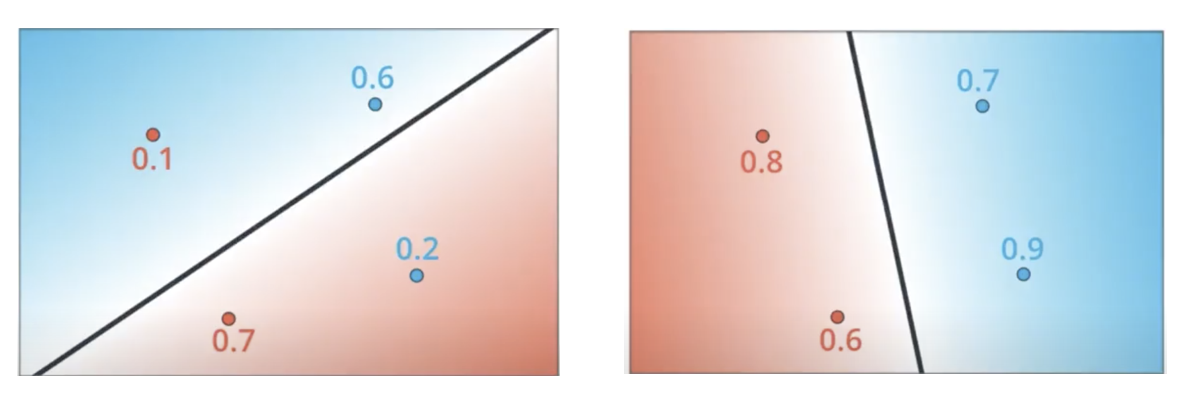• Note that some literature in the field denotes the prediction as $$\hat{y}$$ so the same equation then becomes:
$\text {CrossEntropyLoss}=-\left(y_{i} \log \left(\hat{y}_{i}\right)+\left(1-y_{i}\right) \log \left(1-\hat{y}_{i}\right)\right)$
• Below we see the formula for when our number of classes $$M$$ is greater than 2.
$\text {CrossEntropyLoss}=-\sum_{c=1}^{M} y_{o, c} \log \left(p_{o, c}\right)$
• Note the variables and their meanings:
• $$M$$: The number of classes or output we want to predict (Red, Black, Blue)
• $$y$$: 0 or 1, binary indicator if the class $$c$$ is the correct classification for observation $$o$$
• $$p$$: predicted probability

### Kullback–Leibler (KL) Divergence

• The Kullback–Leibler divergence, denoted $$D_{\text{KL}}(P\parallel Q)}D_{\text{KL}}(P\parallel Q$$, is a type of statistical distance: a measure of how one probability distribution $$P$$ is different from a second, reference probability distribution $$Q$$.
• A simple interpretation of the KL divergence of $$P$$ from $$Q$$ is the expected excess surprise from using $$Q$$ as a model when the actual distribution is $$P$$.
• Note that KL divergence is commonly used as a difference (loss) and not a metric since it is not symmetric in the two distributions, i.e., $$D_{\mathrm{KL}}(P \| Q) \neq D_{\mathrm{KL}}(Q \| P)$$.

• For discrete probability distributions $$P$$ and $$Q$$ defined on the same probability space, $$\mathcal{X}$$, the relative entropy from $$Q$$ to $$P$$ is defined to be:

$D_{\mathrm{KL}}(P \| Q)=\sum_{x \in \mathcal{X}} P(x) \log \left(\frac{P(x)}{Q(x)}\right) .$
• which is equivalent to
$D_{\mathrm{KL}}(P \| Q)=-\sum_{x \in \mathcal{X}} P(x) \log \left(\frac{Q(x)}{P(x)}\right)$
• In other words, it is the expectation of the logarithmic difference between the probabilities $$P$$ and $$Q$$, where the expectation is taken using the probabilities $$P$$.
• Kullback-Leibler Divergence Explained offers a walk-through of KL divergence using an example.

#### KL divergence vs. Cross-entropy loss

• Explanation 1:

• You will need some conditions to claim the equivalence between minimizing cross entropy and minimizing $$\mathrm{KL}$$ divergence. I will put your question under the context of classification problems using cross entropy as loss functions.

• Let us first recall that entropy is used to measure the uncertainty of a system, which is defined as,

$S(v)=-\sum_i p\left(v_i\right) \log p\left(v_i\right)$
• for $$p\left(v_i\right)$$ as the probabilities of different states $v_i$ of the system. From an information theory point of view, $$S(v)$$ is the amount of information is needed for removing the uncertainty.
• For instance, the event $$I$$ I will die within 200 years is almost certain (we may solve the aging problem for the word almost), therefore it has low uncertainty which requires only the information of the aging problem cannot be solved to make it certain. However, the event $$II$$ I will die within 50 years is more uncertain than event $$I$$, thus it needs more information to remove the uncertainties. Here entropy can be used to quantify the uncertainty of the distribution When will I die?, which can be regarded as the expectation of uncertainties of individual events like $$I$$ and $$II$$.
• Now look at the definition of KL divergence between distributions $$\mathrm{A}$$ and $$\mathrm{B}$$,
$D_{K L}(A \| B)=\sum_i p_A\left(v_i\right) \log p_A\left(v_i\right)-p_A\left(v_i\right) \log p_B\left(v_i\right)$
• where the first term of the right hand side is the entropy of distribution $$A$$, the second term can be interpreted as the expectation of distribution $$\mathrm{B}$$ in terms of $$A$$. And the $$D_{K L}$$ describes how different $$\mathrm{B}$$ is from $$\mathrm{A}$$ from the perspective of $$\mathrm{A}$$. It’s worth of noting $$A$$ usually stands for the data, i.e. the measured distribution, and $$B$$ is the theoretical or hypothetical distribution. That means, you always start from what you observed.

• To relate cross entropy to entropy and KL divergence, we formalize the cross entropy in terms of distributions $$A$$ and $$B$$ as,

$H(A, B)=-\sum_i p_A\left(v_i\right) \log p_B\left(v_i\right)$
• From the definitions, we can easily see,
$H(A, B)=D_{K L}(A \| B)+S_A$
• If $$S_A$$ is a constant, then minimizing $$H(A, B)$$ is equivalent to minimizing $$D_{K L}(A \| B)$$.

• A further question follows naturally as how the entropy can be a constant. In a machine learning task, we start with a dataset (denoted as $$P(\mathcal{D})$$) which represent the problem to be solved, and the learning purpose is to make the model estimated distribution (denoted as $$P(model)$$) as close as possible to true distribution of the problem (denoted as $$P(truth)$$). $$P(truth)$$ is unknown and represented by $$P(\mathcal{D})$$. Therefore in an ideal world, we expect

$P(\text { model }) \approx P(\mathcal{D}) \approx P(\text { truth })$
• and minimize $$D_{K L}(P(\mathcal{D}) \| P(model))$$. And luckily, in practice $$\mathcal{D}$$ is given, which means its entropy $$S(D)$$ is fixed as a constant.
• Explanation 2:

• Considering models usually work with the samples packed in mini-batches, for $$\mathrm{KL}$$ divergence and Cross-Entropy, their relation can be written as:

$H(q, p)=D_{K L}(p, q)+H(p)=-\sum_i p_i \log \left(q_i\right)$
• which gives:

$D_{K L}(p, q)=H(q, p)-H(p)$
• From the equation, we can see that KL divergence can depart into a Cross-Entropy of $$p$$ and $$q$$ ($$KL(p, q)$$, which is the first part), and a global entropy of ground truth $$p$$ ($$H(p)$$, which is the second part).
• In many machine learning projects, mini-batch is involved to expedite training, where the $$p^{\prime}$$ of a minibatch may be different from the global $$p$$. In such a case, Cross-Entropy is relatively more robust in practice while $$\mathrm{KL}$$ divergence needs a more stable $$\mathrm{H}(\mathrm{p})$$ to finish her job.

### Hinge Loss / Multi-class SVM Loss

• The hinge loss is used for “maximum-margin” classification, most notably for support vector machines (SVMs).
• The hinge loss is a convex function, so many of the usual convex optimizers used in machine learning can work with it.
• For an intended output $$t = \pm1$$ and a classifier score y, the hinge loss of the prediction y is defined as:
$\ell(y) = \max(0, 1-t \cdot y)$
• The hinge loss is a specific type of cost function that incorporates a margin or distance from the classification boundary into the cost calculation.
• Even if new observations are classified correctly, they can incur a penalty if the margin from the decision boundary is not large enough. The hinge loss increases linearly.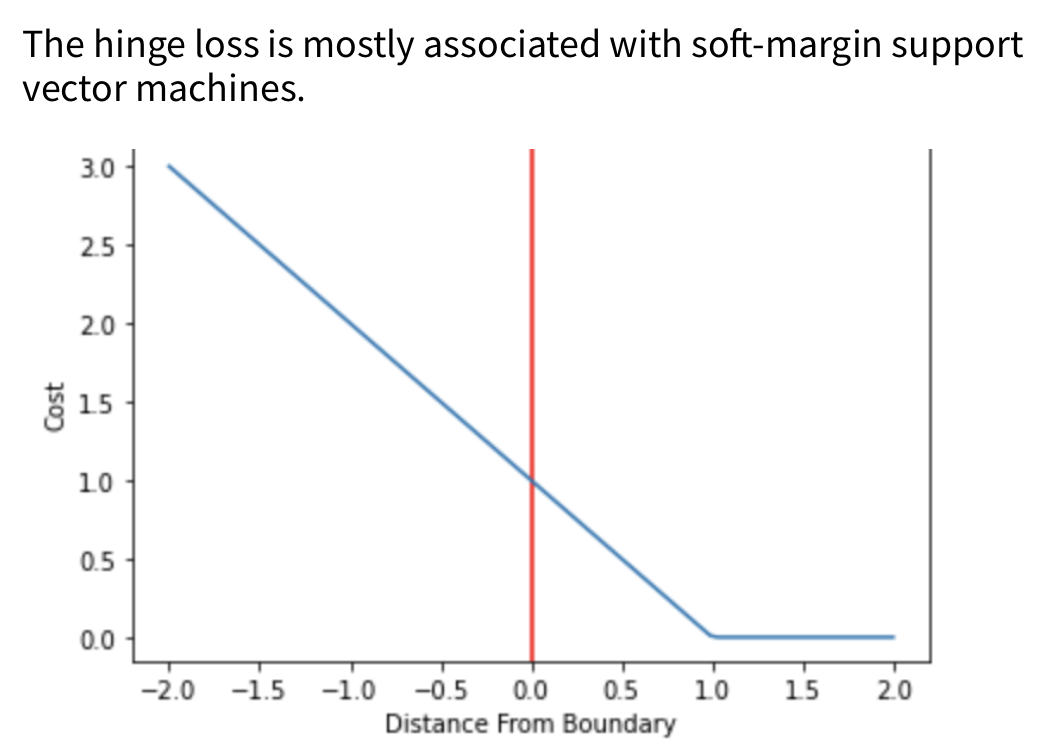### Focal Loss

• Proposed in Focal Loss for Dense Object Detection by Lin et al. in 2017.
• One of the most common choices when training deep neural networks for object detection and classification problems in general.
• Focal loss applies a modulating term to the cross entropy loss in order to focus learning on hard misclassified examples. It is a dynamically scaled cross entropy loss, where the scaling factor decays to zero as confidence in the correct class increases.
$\mathrm{FL}\left(p_{t}\right)=-\left(1-p_{t}\right)^{\gamma} \log \left(p_{t}\right)$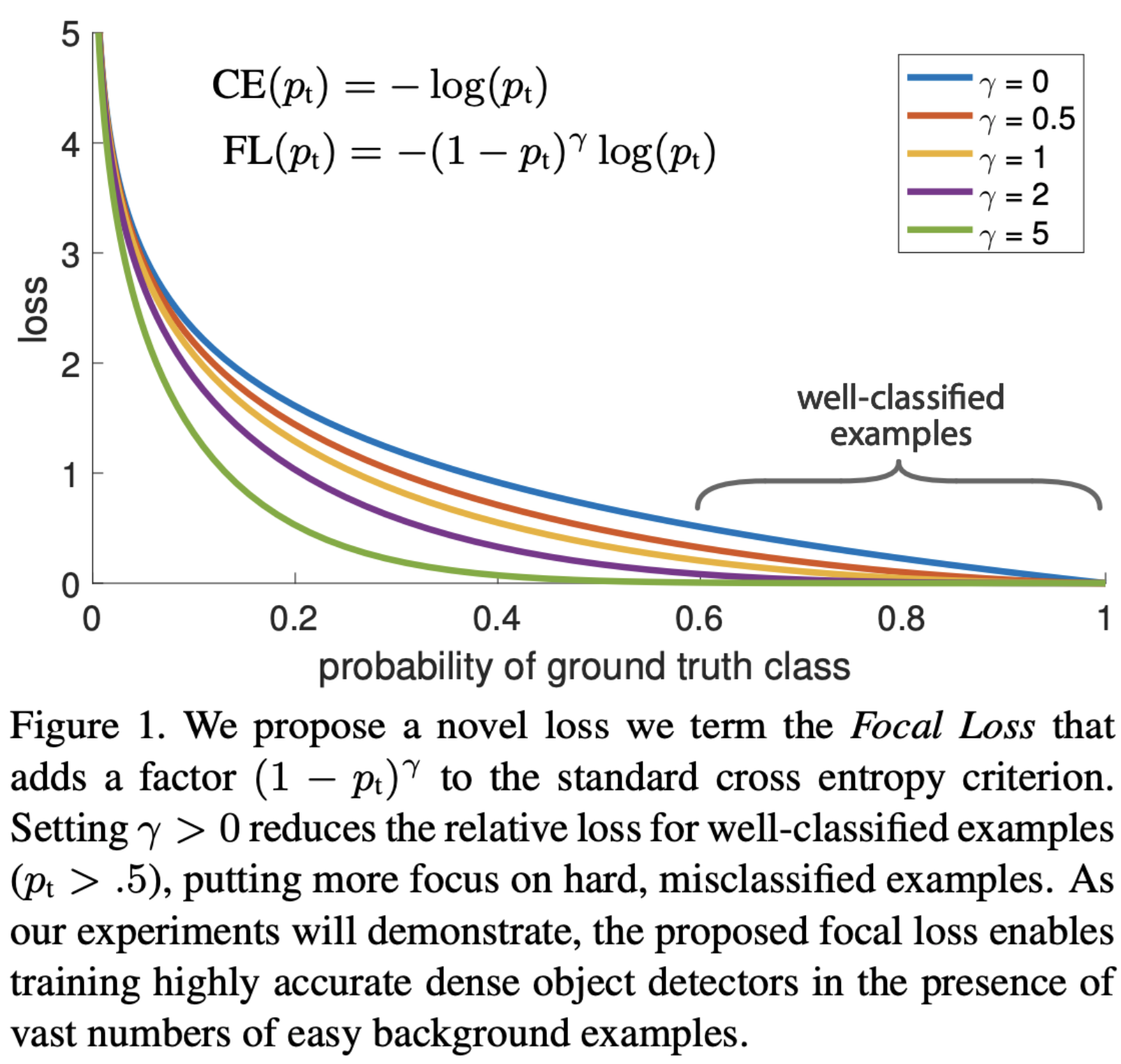### PolyLoss

• Proposed in PolyLoss: A Polynomial Expansion Perspective of Classification Loss Functions by Leng et al. in 2022.
• Cross-entropy loss and focal loss are the most common choices when training deep neural networks for classification problems.
• Generally speaking, however, a good loss function can take on much more flexible forms, and should be tailored for different tasks and datasets.
• PolyLoss is a generalized form of Cross Entropy loss.
• The paper proposes a framework to view and design loss functions as a linear combination of polynomial functions, motivated by how functions can be approximated via Taylor expansion. Under polynomial expansion, focal loss is a horizontal shift of the polynomial coefficients compared to the cross-entropy loss.
• Motivated by this new insight, they explore an alternative dimension, i.e., vertically modify the polynomial coefficients.
$\text { PolyLoss }=\sum_{i=1}^{n} \epsilon_{i} \frac{\left(1-p_{t}\right)^{i}}{i}+C E \text { Loss }$

### Generalized End-to-End Loss

• Proposed in Generalized End-to-End Loss for Speaker Verification by Wan et al. in ICASSP 2018.
• GE2E makes the training of speaker verification models more efficient than our previous tuple-based end-to-end (TE2E) loss function.
• Unlike TE2E, the GE2E loss function updates the network in a way that emphasizes examples that are difficult to verify at each step of the training process.
• Additionally, the GE2E loss does not require an initial stage of example selection.
$L\left(\mathbf{e}_{j i}\right)=-\mathbf{S}_{j i, j}+\log \sum_{k=1}^{N} \exp \left(\mathbf{S}_{j i, k}\right)$

• Proposed in ArcFace: Additive Angular Margin Loss for Deep Face Recognition by Deng et al. in 2018.
• AAM has been predominantly utilized in for face recognition but has recently found applications in other areas such as speaker verification.
• One of the main challenges in feature learning using Deep Convolutional Neural Networks (DCNNs) for large-scale face recognition is the design of appropriate loss functions that enhance discriminative power.
• Centre loss penalises the distance between the deep features and their corresponding class centres in the Euclidean space to achieve intra-class compactness.
• SphereFace assumes that the linear transformation matrix in the last fully connected layer can be used as a representation of the class centres in an angular space and penalises the angles between the deep features and their corresponding weights in a multiplicative way.
• Recently, a popular line of research is to incorporate margins in well-established loss functions in order to maximise face class separability.
• Additive Angular Margin (AAM) Loss (ArcFace) obtains highly discriminative features with a clear geometric interpretation (better than other loss functions) due to the exact correspondence to the geodesic distance on the hypersphere.
• ArcFace consistently outperforms the state-of-the-art and can be easily implemented with negligible computational overhead. We release all refined training data, training codes, pre-trained models and training logs, which will help reproduce the results in this paper.
• Specifically, the proposed ArcFace $$\cos(\theta + m)$$ directly maximises the decision boundary in angular (arc) space based on the L2 normalised weights and features.

$-\frac{1}{N} \sum_{i=1}^{N} \log \frac{e^{S *\left(\cos \left(\theta_{y_{i}}+m\right)\right)}}{e^{s *\left(\cos \left(\theta_{y_{i}}+m\right)\right)}+\sum_{j=1, j \neq y_{i}}^{n} e^{s * \cos \theta_{j}}}$
• where,
• $$\theta_{j}$$ is the angle between the weight $$W_{j}$$ and the feature $$x_{i}$$
• $$s$$: feature scale, the hypersphere radius
• $$m$$: angular margin penalty

### Triplet Loss

• Proposed in FaceNet: A Unified Embedding for Face Recognition and Clustering by Schroff et al. in CVPR 2015.
• Triplet loss was orginally used to learn face recognition of the same person at different poses and angles.
• Triplet loss is a loss function for machine learning algorithms where a reference input (called anchor) is compared to a matching input (called positive) and a non-matching input (called negative).
$\mathcal{J}=\sum_{i=1}^{M} \mathcal{L}\left(A^{(i)}, P^{(i)}, N^{(i)}\right)$
• where,
• $$A$$ is an anchor input
• $$P$$ is a positive input of the same class as {\displaystyle A}A
• $$N$$ is a negative input of a different class from {\displaystyle A}A
• Alpha is a margin between positive and negative pairs
• $$f$$ is an embedding
• Consider the task of training a neural network to recognize faces (e.g. for admission to a high security zone).
• A classifier trained to classify an instance would have to be retrained every time a new person is added to the face database.
• This can be avoided by posing the problem as a similarity learning problem instead of a classification problem.
• Here the network is trained (using a contrastive loss) to output a distance which is small if the image belongs to a known person and large if the image belongs to an unknown person.
• However, if we want to output the closest images to a given image, we would like to learn a ranking and not just a similarity.
• A triplet loss is used in this case.

### InfoNCE Loss

• Proposed in [Contrastive Predictive Coding]((https://arxiv.org/pdf/1807.03748v2.pdf) by van den Oord et al. in 2018.
• InfoNCE, where NCE stands for Noise-Contrastive Estimation, is a type of contrastive loss function used for self-supervised learning.
• The InfoNCE loss, inspired by NCE, uses categorical cross-entropy loss to identify the positive sample amongst a set of unrelated noise samples.
$\mathcal{L}_{\mathrm{N}}=-\underset{X}{\mathbb{E}}\left[\log \frac{f_{k}\left(x_{t+k}, c_{t}\right)}{\sum_{x_{j} \in X} f_{k}\left(x_{j}, c_{t}\right)}\right]$

### Dice Loss

$D=\frac{2 \sum_{i}^{N} p_{i} g_{i}}{\sum_{i}^{N} p_{i}^{2}+\sum_{i}^{N} g_{i}^{2}}$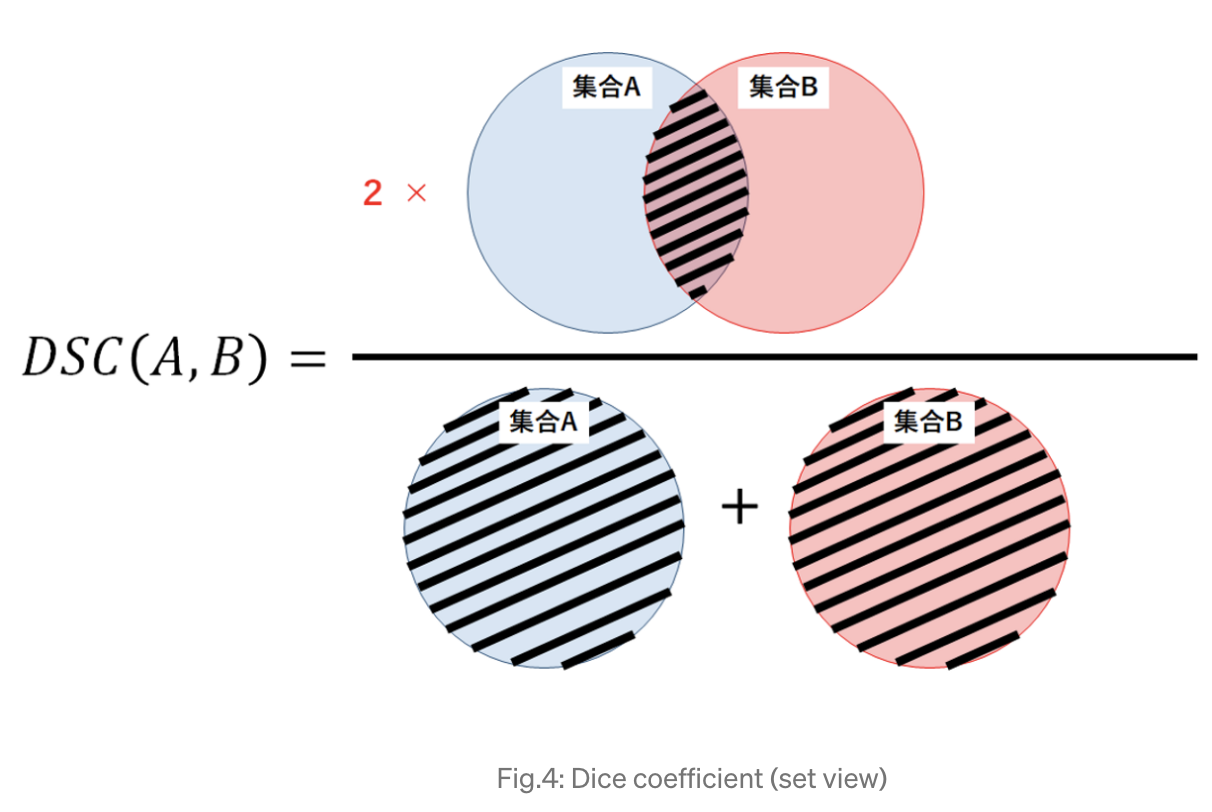• The image above another view of the Dice coefficient mentioned above, from the perspective of set theory, in which the Dice coefficient (DSC) is a measure of overlap between two sets.
• For example, if two sets A and B overlap perfectly, DSC gets its maximum value to 1. Otherwise, DSC starts to decrease, getting to its minimum value to 0 if the two sets don ‘t overlap at all.
• Therefore, the range of DSC is between 0 and 1, the larger the better. Thus we can use 1-DSC as Dice loss to maximize the overlap between two sets.

### Margin Ranking Loss

• Proposed in Adaptive Margin Ranking Loss for Knowledge Graph Embeddings via a Correntropy Objective Function by Nayyeri et al. in 2019.
• As the name suggests, Margin Ranking Loss (MRL) is used for ranking problems.
• MRL calculates the loss provided there are inputs $$X1$$, $$X2$$, as well as a label tensor, $$y$$ containing 1 or -1.
• When the value of $$y$$ is 1 the first input will be assumed as the larger value and will be ranked higher than the second input.
• Similarly, if $$y=-1$$, the second input will be ranked as higher. It is mostly used in ranking problems.
$\mathcal{L}=\sum_{(h, r, t) \in S^{+}} \sum_{\left(h^{\prime}, r^{\prime}, t^{\prime}\right) \in S^{-}}\left[f_{r}(h, t)+\gamma-f_{r}\left(h^{\prime}, t^{\prime}\right)\right]_{+}$
first_input = torch.randn(3, requires_grad=True)
target = torch.randn(3).sign()

ranking_loss = nn.MarginRankingLoss()
output = ranking_loss(first_input, Second_input, target)
output.backward()
print('input one: ', first_input)
print('input two: ', Second_input)
print('target: ', target)
print('output: ', output)


### Contrastive Loss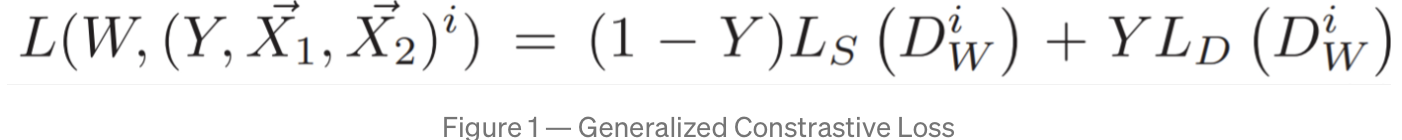• Proposed in Dimensionality Reduction by Learning an Invariant Mapping by Hadsell et al. (with Yann LeCun) in IEEE CVPR 2006.
• Contrastive loss is a distance-based loss as opposed to more conventional error-prediction losses. This loss is used to learn embeddings in which two “similar” points have a low Euclidean distance and two “dissimilar” points have a large Euclidean distance.
• Contrastive loss takes the output of the network for a positive example and calculates its distance to an example of the same class and contrasts that with the distance to negative examples.
• Two samples are either similar or dissimilar. This binary similarity can be determined using several approaches:
• In this work, the $$N$$ closest neighbors of a sample in input space (e.g. pixel space) are considered similar; all others are considered dissimilar. (This approach yields a smooth latent space; e.g. the latent vectors for two similar views of an object are close)
• To the group of similar samples to a sample, we can add transformed versions of the sample (e.g. using data augmentation). This allows the latent space to be invariant to one or more transformations.
• We can use a manually obtained label determining if two samples are similar. (For example, we could use the class label. However, there can be cases where two samples from the same class are relatively dissimilar, or where two samples from different classes are relatively similar. Using classes alone does not encourage a smooth latent space.)
• Put simply, clusters of points belonging to the same class are pulled together in embedding space, while simultaneously pushing apart clusters of samples from different classes. In other words, contrastive loss calculates the distance between positive example (example of the same class) and negative example (example not of the same class). So loss can be expected to be low if the positive examples are encoded (in this embedding space) to similar examples and the negative ones are further away encoded to different representations. This behavior is illustrated in the image below: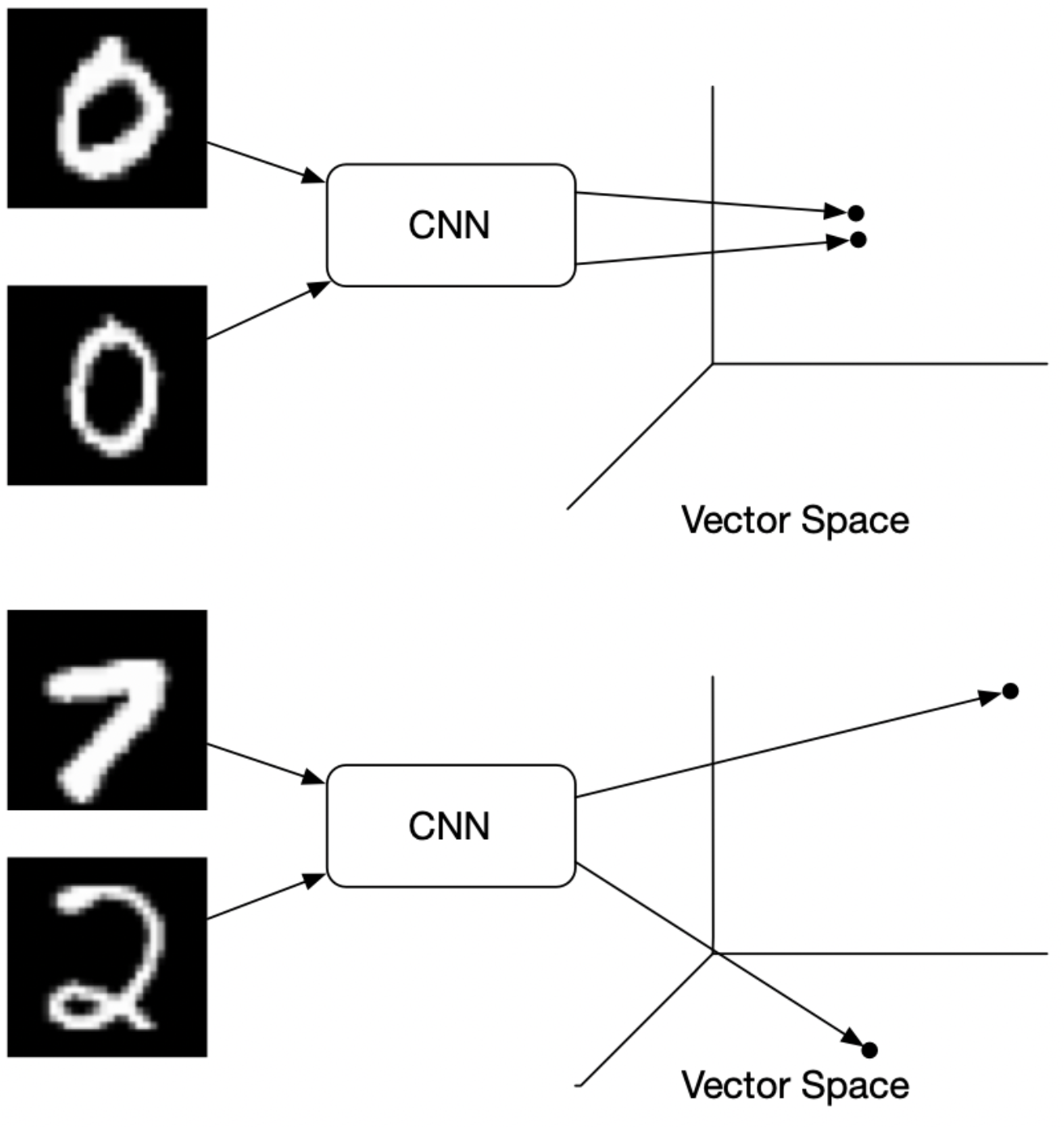• Formally, if we consider $\vec{X}$ as the input data and $G_W(\vec{X})$ the output of a neural network, the interpoint distance is given by,
$D_W\left(\vec{X}_1, \vec{X}_2\right)=\left\|G_W\left(\vec{X}_1\right)-G_W\left(\vec{X}_2\right)\right\|_2$
• The contrastive loss is simply,

\begin{aligned} \mathcal{L}(W) &=\sum_{i=1}^P L\left(W,\left(Y, \vec{X}_1, \vec{X}_2\right)^i\right) \\ L\left(W,\left(Y, \vec{X}_1, \vec{X}_2\right)^i\right) &=(1-Y) L_S\left(D_W^i\right)+Y L_D\left(D_W^i\right) \end{aligned}
• where $Y=0$ when $X_1$ and $X_2$ are similar and $Y=1$ otherwise, and $L_S$ is a loss for similar points and $L_D$ is a loss for dissimilar points.
• More formally, the contrastive loss is given by,

\begin{aligned} &L\left(W, Y, \vec{X}_1, \vec{X}_2\right)= \\ &\quad(1-Y) \frac{1}{2}\left(D_W\right)^2+(Y) \frac{1}{2}\left\{\max \left(0, m-D_W\right)\right\}^2 \end{aligned}
• where $$m$$ is a predefined margin.
• The gradient is given by the simple equations:

$\begin{gathered} \frac{\partial L_S}{\partial W}=D_W \frac{\partial D_W}{\partial W} \\ \frac{\partial L_D}{\partial W}=-\left(m-D_W\right) \frac{\partial D_W}{\partial W} \end{gathered}$
• Contrastive Loss is often used in image retrieval tasks to learn discriminative features for images. During training, an image pair is fed into the model with their ground truth relationship: equals 1 if the two images are similar and 0 otherwise. The loss function for a single pair is:

$y d^2+(1-y) \max (\operatorname{margin}-d, 0)^2$
• where $$d$$ is the Euclidean distance between the two image features (suppose their features are $$f_1$$ and $$f_2$$): $$d=\left \mid f_1-f_2\right \mid_2$$. The $$margin$$ term is used to “tighten” the constraint: if two images in a pair are dissimilar, then their distance should be at least $$margin$$, or a loss will be incurred.
• Shown below are the results from the paper which are quite convincing:• Note that while this is one of the earliest of the contrastive losses, this is not the only one. For instance, the contrastive loss used in SimCLR is quite different.

### Multiple Negative Ranking Loss

• Proposed in Efficient Natural Language Response Suggestion for Smart Reply by Henderson et al. from Google in 2017.
• Multiple Negative Ranking (MNR) Loss is a great loss function if you only have positive pairs, for example, only pairs of similar texts like pairs of paraphrases, pairs of duplicate questions, pairs of (query, response), or pairs of (source_language, target_language).
• This loss function works great to train embeddings for retrieval setups where you have positive pairs (e.g. (query, relevant_doc)) as it will sample n-1 negative docs in each batch randomly.The performance usually increases with increasing batch sizes.
• This is because with MNR loss, we drop all rows with neutral or contradiction labels — keeping only the positive entailment pairs (source).
• Models trained with MNR loss outperform those trained with softmax loss in high-performing sentence embeddings problems.
• Below is a code sample referenced from sbert.net:
from sentence_transformers import SentenceTransformer, losses, InputExample

model = SentenceTransformer('distilbert-base-uncased')
train_examples = [InputExample(texts=['Anchor 1', 'Positive 1']),
InputExample(texts=['Anchor 2', 'Positive 2'])]
train_loss = losses.MultipleNegativesRankingLoss(model=model)


## Regression Loss Functions

### Mean Average Error or L1 loss

• As the name suggests, MAE takes the average sum of the absolute differences between the actual and the predicted values.
• Regression problems may have variables that are not strictly Gaussian in nature due to the presence of outliers (values that are very different from the rest of the data).
• Mean Absolute Error would be an ideal option in such cases because it does not take into account the direction of the outliers (unrealistically high positive or negative values).

$M A E=\frac{1}{m} \sum_{i=1}^{m}\left|h\left(x^{(i)}\right)-y^{(i)}\right|$
• where,
• MAE: mean absolute error
• $$\mathrm{m}$$: number of samples
• $$x^{(i)}$$: $$i^{th}$$ sample from dataset
• $$h\left(x^{(i)}\right)$$: prediction for i-th sample (thesis)
• $$y^{(i)}$$: ground truth label for $$\mathrm{i}$$-th sample
• A quick note here on L1 and L2, these are both used for regularization.
• L1 Loss Function is used to minimize the error which is the sum of the all the absolute differences between the true value and the predicted value.
• L1 is not affected by outliers and thus is preferrable if the dataset contains outliers.

### Mean Squared Error or L2 loss

$M S E=\frac{1}{m} \sum_{i=1}^{m}\left(y^{(i)}-\hat{y}^{(i)}\right)^{2}$
• where,
• MSE: mean square error
• $$\mathrm{m}$$: number of samples
• $$y^{(i)}$$: ground truth label for i-th sample
• $$\hat{y}^{(i)}$$: predicted label for i-th sample
• Mean Squared Error is the average of the squared differences between the actual and the predicted values.
• L2 Loss Function is used to minimize the error which is the sum of the all the squared differences between the true value and the predicted value. It is also the more preferred loss function compared to L1.
• However, when outliers are present in the dataset, L2 will not perform as well because the squared differences will lead to a much larger error.

### Huber Loss / Smooth Mean Absolute Error

• Huber loss is a loss function used in regression, that is less sensitive to outliers in data than the squared error loss.
• Huber loss is the combination of MSE and MAE. It takes the good properties of both the loss functions by being less sensitive to outliers and differentiable at minima.
• When the error is smaller, the MSE part of the Huber is utilized and when the error is large, the MAE part of Huber loss is used.
• A new hyper-parameter $$\delta$$ is introduced which tells the loss function where to switch from MSE to MAE.
• Additional $$\delta$$ terms are introduced in the loss function to smoothen the transition from MSE to MAE.
• The Huber loss function describes the penalty incurred by an estimation procedure $$f$$. Huber loss defines the loss function piecewise by:
$L_{\delta}(a)= \begin{cases}\frac{1}{2} a^{2} & \text { for }|a| \leq \delta \\ \delta \cdot\left(|a|-\frac{1}{2} \delta\right), & \text { otherwise }\end{cases}$
• This function is quadratic for small values of $$a$$, and linear for large values, with equal values and slopes of the different sections at the two points where $$\|a\|=\delta$$. The variable a often refers to the residuals, that is to the difference between the observed and predicted values $$a=y-f(x)$$, so the former can be expanded to:
$L_{\delta}(y, f(x))= \begin{cases}\frac{1}{2}(y-f(x))^{2} & \text { for }|y-f(x)| \leq \delta \\ \delta \cdot\left(|y-f(x)|-\frac{1}{2} \delta\right), & \text { otherwise }\end{cases}$
• The below diagram (source) compares Huber loss with squared loss and absolute loss: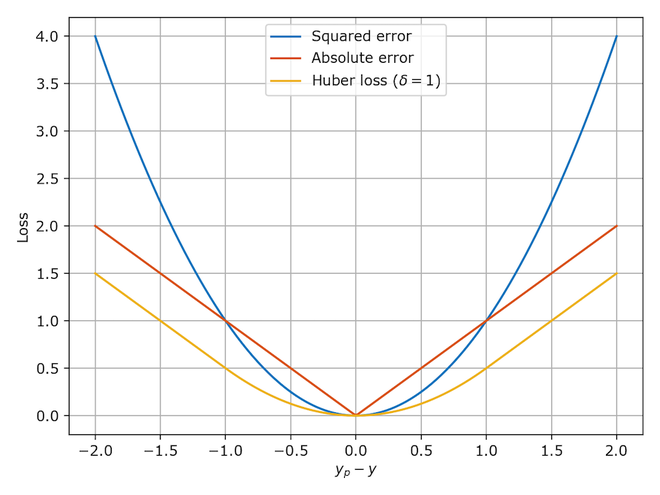@article{Chadha2020DistilledLossFunctions,